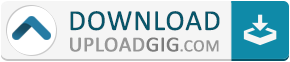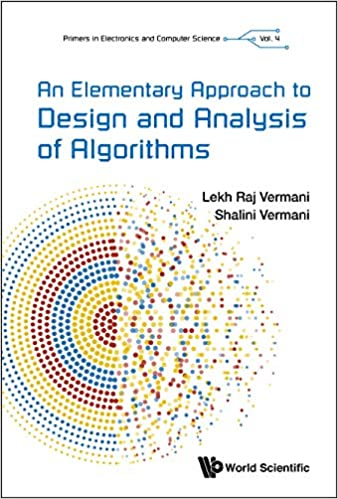Do not remember me!

Categories» » » An Elementary Approach to Design and Analysis of Algorithms (Primers in Electronics and Computer Science)

An Elementary Approach to Design and Analysis of Algorithms (Primers in Electronics and Computer Science)English | 2019 | ISBN: ‎ 1786346753 | 628 pages | True EPUB | 31.09 MB

'The book under review is an interesting elaboration that fills the gaps in libraries for concisely written and student-friendly books about essentials in computer science … I recommend this book for anyone who would like to study algorithms, learn a lot about computer science or simply would like to deepen their knowledge … The book is written in very simple English and can be understood even by those with limited knowledge of the English language. It should be emphasized that, despite the fact that the book consists of many examples, mathematical formulas and theorems, it is very hard to find any mistakes, errors or typos.'zbMATHIn computer science, an algorithm is an unambiguous specification of how to solve a class of problems. Algorithms can perform calculation, data processing and automated reasoning tasks.As an effective method, an algorithm can be expressed within a finite amount of space and time and in a well-defined formal language for calculating a function. Starting from an initial state and initial input (perhaps empty), the instructions describe a computation that, when executed, proceeds through a finite number of well-defined successive states, eventually producing 'output' and terminating at a final ending state. The transition from one state to the next is not necessarily deterministic; some algorithms, known as randomized algorithms, incorporate random input.This book introduces a set of concepts in solving problems computationally such as Growth of Functions; Backtracking; Divide and Conquer; Greedy Algorithms; Dynamic Programming; Elementary Graph Algorithms; Minimal Spanning Tree; Single-Source Shortest Paths; All Pairs Shortest Paths; Flow Networks; Polynomial Multiplication, to ways of solving NP-Complete Problems, supported with comprehensive, and detailed problems and solutions, making it an ideal resource to those studying computer science, computer engineering and information technology.Name:* E-Mail: Security Code: *